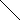# MINLPLib

### A Library of Mixed-Integer and Continuous Nonlinear Programming Instances

#### Instance ball_mk3_20

```A simple MINLP with a feasible set described by a ball.
The basic model over which these variations are made is:
min  sum_i=1^n x_i
s.t. sum_i=1^n (x_i - 0.5)^2 <= (n-1)/4
x integer between -1 and 1.
Obvisouly, this problem is infeasible and has no solution.
It can be shown that any outer-approximation based method will need 2^n linear inequalities to show infeasibility, see reference.
In this instance, the ball is an empty ellipse, but the quadratic form is still diagonal.```
 Formatsⓘ ams gms lp mod nl osil pip py Primal Bounds (infeas ≤ 1e-08)ⓘ Other points (infeas > 1e-08)ⓘ Dual Boundsⓘ -21.00000000 (ALPHAECP)inf (ANTIGONE)inf (BARON)-9.00843129 (BONMIN)inf (COUENNE)inf (CPLEX)inf (GUROBI)inf (LINDO)inf (SCIP)inf (SHOT) Referencesⓘ Hijazi, Hassan, Bonami, Pierre, and Ouorou, Adam, An Outer-Inner Approximation for Separable Mixed-Integer Nonlinear Programs, INFORMS Journal on Computing, 26:1, 2014, 31-44. Sourceⓘ Pierre Bonami Applicationⓘ Geometry Added to libraryⓘ 11 Sep 2017 Problem typeⓘ IQCP #Variablesⓘ 20 #Binary Variablesⓘ 0 #Integer Variablesⓘ 20 #Nonlinear Variablesⓘ 20 #Nonlinear Binary Variablesⓘ 0 #Nonlinear Integer Variablesⓘ 20 Objective Senseⓘ min Objective typeⓘ linear Objective curvatureⓘ linear #Nonzeros in Objectiveⓘ 20 #Nonlinear Nonzeros in Objectiveⓘ 0 #Constraintsⓘ 1 #Linear Constraintsⓘ 0 #Quadratic Constraintsⓘ 1 #Polynomial Constraintsⓘ 0 #Signomial Constraintsⓘ 0 #General Nonlinear Constraintsⓘ 0 Operands in Gen. Nonlin. Functionsⓘ Constraints curvatureⓘ convex #Nonzeros in Jacobianⓘ 20 #Nonlinear Nonzeros in Jacobianⓘ 20 #Nonzeros in (Upper-Left) Hessian of Lagrangianⓘ 20 #Nonzeros in Diagonal of Hessian of Lagrangianⓘ 20 #Blocks in Hessian of Lagrangianⓘ 20 Minimal blocksize in Hessian of Lagrangianⓘ 1 Maximal blocksize in Hessian of Lagrangianⓘ 1 Average blocksize in Hessian of Lagrangianⓘ 1.0 #Semicontinuitiesⓘ 0 #Nonlinear Semicontinuitiesⓘ 0 #SOS type 1ⓘ 0 #SOS type 2ⓘ 0 Minimal coefficientⓘ 8.8922e-03 Maximal coefficientⓘ 1.0000e+00 Infeasibility of initial pointⓘ 0.0001 Sparsity JacobianⓘSparsity Hessian of Lagrangianⓘ```\$offlisting
*
*  Equation counts
*      Total        E        G        L        N        X        C        B
*          2        1        0        1        0        0        0        0
*
*  Variable counts
*                   x        b        i      s1s      s2s       sc       si
*      Total     cont   binary  integer     sos1     sos2    scont     sint
*         21        1        0       20        0        0        0        0
*  FX      0
*
*  Nonzero counts
*      Total    const       NL      DLL
*         41       21       20        0
*
*  Solve m using MINLP minimizing objvar;

Variables  objvar,i2,i3,i4,i5,i6,i7,i8,i9,i10,i11,i12,i13,i14,i15,i16,i17,i18
,i19,i20,i21;

Integer Variables  i2,i3,i4,i5,i6,i7,i8,i9,i10,i11,i12,i13,i14,i15,i16,i17,i18
,i19,i20,i21;

Equations  e1,e2;

e1..    objvar + i2 + i3 + i4 + i5 + i6 + i7 + i8 + i9 + i10 + i11 + i12 + i13
+ i14 + i15 + i16 + i17 + i18 + i19 + i20 + i21 =E= 0;

e2.. 0.0560877535068921*sqr(i20) - 0.0560877535068921*i20 + 0.0234361418326102*
sqr(i19) - 0.0234361418326102*i19 + 0.0804348252437088*sqr(i18) -
0.0804348252437088*i18 + 0.083180630465482*sqr(i17) - 0.083180630465482*
i17 + 0.0168840147598981*sqr(i16) - 0.0168840147598981*i16 +
0.0642555768399037*sqr(i15) - 0.0642555768399037*i15 + 0.0515967329760445*
sqr(i14) - 0.0515967329760445*i14 + 0.0291406913653282*sqr(i13) -
0.0291406913653282*i13 + 0.0358736092274657*sqr(i12) - 0.0358736092274657*
i12 + 0.0403619397376071*sqr(i11) - 0.0403619397376071*i11 +
0.0604484044580273*sqr(i10) - 0.0604484044580273*i10 + 0.0534869191762675*
sqr(i9) - 0.0534869191762675*i9 + 0.0820022096762534*sqr(i8) -
0.0820022096762534*i8 + 0.0369575272885052*sqr(i7) - 0.0369575272885052*i7
+ 0.00889217633273991*sqr(i6) - 0.00889217633273991*i6 +
0.0674863595874412*sqr(i5) - 0.0674863595874412*i5 + 0.0436989257405813*
sqr(i4) - 0.0436989257405813*i4 + 0.0218982155241878*sqr(i3) -
0.0218982155241878*i3 + 0.0699896991762921*sqr(i2) - 0.0699896991762921*i2
+ 0.0738876470847639*sqr(i21) - 0.0738876470847639*i21
=L= -9.99999999999335E-5;

* set non-default bounds
i2.lo = -1; i2.up = 2;
i3.lo = -1; i3.up = 2;
i4.lo = -1; i4.up = 2;
i5.lo = -1; i5.up = 2;
i6.lo = -1; i6.up = 2;
i7.lo = -1; i7.up = 2;
i8.lo = -1; i8.up = 2;
i9.lo = -1; i9.up = 2;
i10.lo = -1; i10.up = 2;
i11.lo = -1; i11.up = 2;
i12.lo = -1; i12.up = 2;
i13.lo = -1; i13.up = 2;
i14.lo = -1; i14.up = 2;
i15.lo = -1; i15.up = 2;
i16.lo = -1; i16.up = 2;
i17.lo = -1; i17.up = 2;
i18.lo = -1; i18.up = 2;
i19.lo = -1; i19.up = 2;
i20.lo = -1; i20.up = 2;
i21.lo = -1; i21.up = 2;

Model m / all /;

m.limrow=0; m.limcol=0;
m.tolproj=0.0;

\$if NOT '%gams.u1%' == '' \$include '%gams.u1%'

\$if not set MINLP \$set MINLP MINLP
Solve m using %MINLP% minimizing objvar;

```

Last updated: 2023-03-21 Git hash: 0a21635c